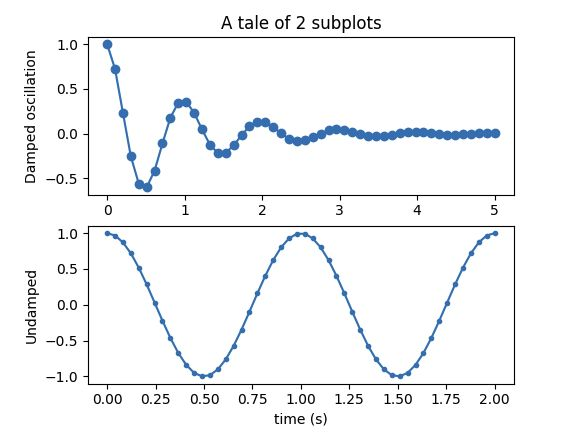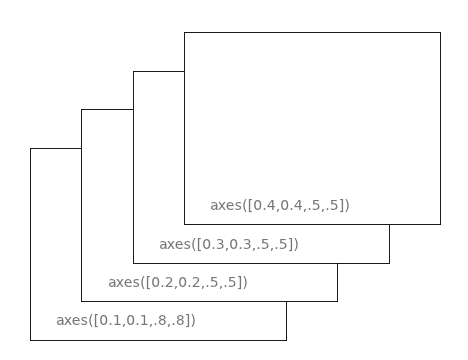# 14.4 【可视化之matplotlib】难点：子图与子区¶

## 1. 子区¶

```plt.subplot(2, 2, 1)
```

```# 第一种写法
ax = plt.subplot(2, 2, 1)

# 第二种写法
ax = plt.subplot2grid((2, 2), (0, 0))

# 第三种写法: GridSpec
import matplotlib.gridspec as gridspec
gs = gridspec.GridSpec(2, 2)
ax = plt.subplot(gs[0, 0])
```

```import numpy as np
import matplotlib.pyplot as plt

def fig(t):
return np.exp(-t) * np.cos(2*np.pi*t)

t1 = np.arange(0.0, 5.0, 0.1)
t2 = np.arange(0.0, 5.0, 0.02)

plt.figure(1)
# 等同于 plt.subplot(2, 1，1)
plt.subplot(211)
plt.plot(t1, fig(t1), 'bo', t2, fig(t2), 'k')

# 等同于 plt.subplot(2, 1，2)
plt.subplot(212)
plt.plot(t2, np.cos(2*np.pi*t2), 'r--')
plt.show()
```## 2. 子图¶

```axes1 = plt.subplot2grid((3, 3), (0, 0), colspan=3)
axes2 = plt.subplot2grid((3, 3), (1, 0), colspan=2)
axes3 = plt.subplot2grid((3, 3), (1, 2), rowspan=2)
axes4 = plt.subplot2grid((3, 3), (2, 0))
axes5 = plt.subplot2grid((3, 3), (2, 1))
```

```import matplotlib.gridspec as gridspec
gs = gridspec.GridSpec(3, 3)
ax1 = plt.subplot(gs[0, :])
ax2 = plt.subplot(gs[1, :-1])
ax3 = plt.subplot(gs[1:, -1])
ax4 = plt.subplot(gs[-1, 0])
ax5 = plt.subplot(gs[-1, -2])
``````import numpy as np
import matplotlib.pyplot as plt

def f(t):
return np.exp(-t) * np.cos(2*np.pi*t)

t1 = np.arange(0.0, 3.0, 0.01)

ax1 = plt.subplot(212)
ax1.margins(0.05)           # Default margin is 0.05, value 0 means fit
ax1.plot(t1, f(t1), 'k')

ax2 = plt.subplot(221)
ax2.margins(2, 2)           # Values >0.0 zoom out
ax2.plot(t1, f(t1), 'r')
ax2.set_title('Zoomed out')

ax3 = plt.subplot(222)
ax3.margins(x=0, y=-0.25)   # Values in (-0.5, 0.0) zooms in to center
ax3.plot(t1, f(t1), 'g')
ax3.set_title('Zoomed in')

plt.show()
````left` 是指，离左边界的距离。 `bottom` 是指，离底边的距离。 `width` 是指，子图的宽度。 `height` 是指，子图的高度。

```import matplotlib.pyplot as plt
import numpy as np

# Fixing random state for reproducibility
np.random.seed(19680801)

# create some data to use for the plot
dt = 0.001
t = np.arange(0.0, 10.0, dt)
r = np.exp(-t[:1000] / 0.05)  # impulse response
x = np.random.randn(len(t))
s = np.convolve(x, r)[:len(x)] * dt  # colored noise

# the main axes is subplot(111) by default
plt.plot(t, s)
plt.axis([0, 1, 1.1 * np.min(s), 2 * np.max(s)])
plt.xlabel('time (s)')
plt.ylabel('current (nA)')
plt.title('Gaussian colored noise')

# this is an inset axes over the main axes
a = plt.axes([.65, .6, .2, .2], facecolor='k')
n, bins, patches = plt.hist(s, 400, density=True)
plt.title('Probability')
plt.xticks([])
plt.yticks([])

# this is another inset axes over the main axes
b = plt.axes([0.2, 0.6, .2, .2], facecolor='k')
plt.plot(t[:len(r)], r)
plt.title('Impulse response')
plt.xlim(0, 0.2)
plt.xticks([])
plt.yticks([])

plt.show()
```